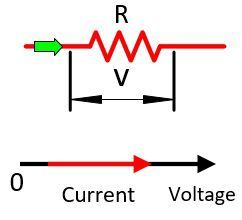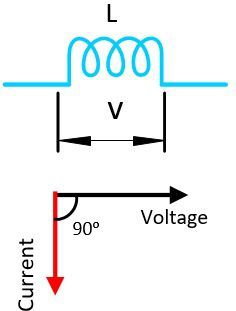# Power in AC Circuit

The power of three-phase AC circuit is used in large industries for running the heavy machines. The power of single-phase AC circuit is used for operating the small household devices. The magnitude of three phase ac circuit power is three times more than the power of the single phase circuit.

Consider if P is the power of the single phase circuit then the 3P is the power of the three-phase balanced ac circuit. The power of the unbalanced three-phase circuit is known by adding the power of the individual phase.

## How to Calculate the Power of the AC circuit?

In dc circuit the value of voltage and current becomes constant. But in AC circuit the instantaneous value of current and voltage flows and hence the power of the supply are continuously varying with the time. So different methods are used for measuring the power of ac and dc circuit.

The instantaneous power of the ac circuit changes continuously when their voltage and current changes. The instantaneous power is the power of the circuit at the specific instant of time. Let p be the power at any instant, v is the voltage and ‘i’ be the current of the circuit.If the sinusoidal current and voltage flow through the ac circuit and the current is lagging behind the voltage by an angle of Φ, in that case, the value of the instantaneous power is given by the expressionIf the voltage and current are out of phase to each other, then the value of current and voltage becomes,The instantaneous power of the circuit is expressed asLet, Θ = ωt

The term cosΦ in the given expression shows that the wattmeter is required for measuring the power of the AC circuit.

## How Power of the AC circuit depends on Power Factor?

The power factor determines the total useful power flows in the circuit. For understanding, the role of power factor in the ac circuit consider the three conditions.

### AC Power in a Purely Resistive Circuit

The resistor is an electrical component which consumed the electrical power of the ac circuit.In a purely resistive circuit, the current flows through the resistor is in phase with the supply voltage, i.e., the waves form of the voltage and current is in phase with each other. The zero-degree phase difference occurs between the waveform of voltage and current.### AC power in a Purely Inductive Circuit

In the purely inductive circuit, the value of voltage and current are not in phase with each other.The voltage and current rise and fall together at the phase shift of 90º. The expression gives the power of the purely inductive circuit.The above equation shows that the inductor neither consumes nor dissipates any electrical power.

### AC Power in a Purely Capacitive Circuit

In the purely capacitive ac circuit, the waveform of voltage and current are out of phase to each other.The current of the circuit is leading with their voltage by an angle of 90º. In spite of dissipating the power the capacitor stores the electrical energy.